SSC CGL Previous Year Questions: Average- 2

# SSC CGL Previous Year Questions: Average- 2 - SSC CGL

Test Description

## 40 Questions MCQ Test SSC CGL (Tier - 1) - Previous Year Papers (Topic Wise) - SSC CGL Previous Year Questions: Average- 2

SSC CGL Previous Year Questions: Average- 2 for SSC CGL 2023 is part of SSC CGL (Tier - 1) - Previous Year Papers (Topic Wise) preparation. The SSC CGL Previous Year Questions: Average- 2 questions and answers have been prepared according to the SSC CGL exam syllabus.The SSC CGL Previous Year Questions: Average- 2 MCQs are made for SSC CGL 2023 Exam. Find important definitions, questions, notes, meanings, examples, exercises, MCQs and online tests for SSC CGL Previous Year Questions: Average- 2 below.
Solutions of SSC CGL Previous Year Questions: Average- 2 questions in English are available as part of our SSC CGL (Tier - 1) - Previous Year Papers (Topic Wise) for SSC CGL & SSC CGL Previous Year Questions: Average- 2 solutions in Hindi for SSC CGL (Tier - 1) - Previous Year Papers (Topic Wise) course. Download more important topics, notes, lectures and mock test series for SSC CGL Exam by signing up for free. Attempt SSC CGL Previous Year Questions: Average- 2 | 40 questions in 40 minutes | Mock test for SSC CGL preparation | Free important questions MCQ to study SSC CGL (Tier - 1) - Previous Year Papers (Topic Wise) for SSC CGL Exam | Download free PDF with solutions
 1 Crore+ students have signed up on EduRev. Have you?
SSC CGL Previous Year Questions: Average- 2 - Question 1

### At an average of 80 km/hr Shatabdi Express reaches Ranchi from Kolkata in 7 hrs. Then the distance between Kolkata and Ranchi is        (SSC CGL 1st Sit. 2016)

Detailed Solution for SSC CGL Previous Year Questions: Average- 2 - Question 1

Distance = Speed × Time = 80 × 7 = 560 km

SSC CGL Previous Year Questions: Average- 2 - Question 2

### The average of 100 observations was calculated as 35.It was found later, that one of the observation was misread as 83 instead of 53. The correct average is:        (SSC CGL 1st Sit. 2016)

Detailed Solution for SSC CGL Previous Year Questions: Average- 2 - Question 2

Average of difference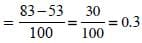Correct Average = 35 – 0 × 3 = 34.7

SSC CGL Previous Year Questions: Average- 2 - Question 3

### The  average of 13 results is 70. The average of first seven is 65 and that of the last seven is 75, the seventh result is :                 (SSC CHSL 2015)

Detailed Solution for SSC CGL Previous Year Questions: Average- 2 - Question 3

Sum of 13 results = 13 × 70 = 910
Sum of 7 results = 7 × 65 = 455
Sum of last 7 results = 7 × 75 = 525
So, 7th result = (455 + 525) – 910 = 70

SSC CGL Previous Year Questions: Average- 2 - Question 4

The average of some natural numbers is 15. If 30 is added to first number and 5 is subtracted from the last number the average becomes 17.5 then the number of natural numbers is:        (SSC Sub. Ins. 2015)

Detailed Solution for SSC CGL Previous Year Questions: Average- 2 - Question 4

Let number of natural numbers be x
∴ 15x + 30 – 5 = 17.5 x
25 = 2.5x
x = 25/2.5 = 10
So 10 natural numbers are there.

SSC CGL Previous Year Questions: Average- 2 - Question 5

What is the arithmetic mean of first 20 odd natural numbers?        (SSC CGL 1st Sit. 2015)

Detailed Solution for SSC CGL Previous Year Questions: Average- 2 - Question 5

For 20 natural last odd number = 1 + (20 – 1) × 2 = 39
Arithmetic mean of odd number = (n + 1)/2
= 40/2 =20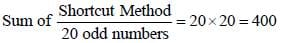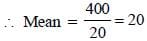SSC CGL Previous Year Questions: Average- 2 - Question 6

3 years ago the average age of a family of 5 members was 17 years. A baby having been born, the average age of the family is the same today. The present age of the baby is       (SSC CHSL 2014)

Detailed Solution for SSC CGL Previous Year Questions: Average- 2 - Question 6

Let total age of family be S years
3 years ago, total age = S – 3 × 5 = S – 15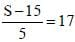S = 17 × 5 + 15 = 100
Let present age of baby be x years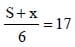100 + x = 17 × 6
x = 102 – 100 = 2 years

SSC CGL Previous Year Questions: Average- 2 - Question 7

The average salary of all the workers in a workshop is ₹ 8,000. The average salary of 7 technicians is ₹ 12,000 and the average salary of the rest is ₹ 6,000. The total number of workers in the workshop is     (SSC CHSL 2014)

Detailed Solution for SSC CGL Previous Year Questions: Average- 2 - Question 7

Let total number of workers be n total salary of all workers = 8000 n
total salary of 7 technicians = 7 × 12000 = 84,000
total salary of remaining workers = (n – 7) × 6000
84000 + (n – 7) × 6000 = 8000 n
84 + 6n – 42 = 8n
42 = 2n
n = 21

SSC CGL Previous Year Questions: Average- 2 - Question 8

A boy found that the average of 20 numbers is 35 when he writes a number '61' instead of '16'. The correct average of 20 numbers is       (SSC Sub. Ins. 2014)

Detailed Solution for SSC CGL Previous Year Questions: Average- 2 - Question 8

Sum of 20 numbers = 20 × 35 = 700
Sum of 20 numbers when 61 is replaced by 16
⇒ 700 – 61 + 16 = 655
Correct average of 20 numbers = 655/20 = 32.75.

SSC CGL Previous Year Questions: Average- 2 - Question 9

The average height of 8 students is 152 cm. Two more students of heights 144 cm and 155 cm join the group. What is the new average height?      (SSC Multitasking 2014)

Detailed Solution for SSC CGL Previous Year Questions: Average- 2 - Question 9

Total height of 8 students = 8 × 152 cm = 1216 cm
Total height of 10 students = 1216 cm + 144 cm + 155 cm = 1515 cm
new average = 1515/10 cm = 151.5cm

SSC CGL Previous Year Questions: Average- 2 - Question 10

What is the Arithmetic mean of the first 'n' natural numbers?      (SSC CGL 1st Sit. 2015)

Detailed Solution for SSC CGL Previous Year Questions: Average- 2 - Question 10

Arithmetic mean of first ‘n’ natural number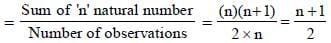SSC CGL Previous Year Questions: Average- 2 - Question 11

The average weight of 15 oarsmen in a boat is increased by 1.6 kg when one of the crew, who weighs 42 kg is replaced by a new man. Find the weight of the new man (in kg).        (SSC CGL 1st Sit. 2015)

Detailed Solution for SSC CGL Previous Year Questions: Average- 2 - Question 11

Let the average weight of 15 Oarsmen at the start = x kg
Let the new man’s weight = y kg
According to question
15x – 42 = 15 (x + 1.6) – y
15x – 42 = 15x + 24 – y
y = 24 + 42 = 66 kg

SSC CGL Previous Year Questions: Average- 2 - Question 12

There are 100 students in 3 sections A, B and C of a class. The average marks of all the 3 sections was 84. The average of B and C was 87.5 and the average marks of A is 70. The number of students in A was          (SSC CGL 2014)

Detailed Solution for SSC CGL Previous Year Questions: Average- 2 - Question 12

Total marks of all three sections = 84 × 100 = 8400
Total marks of (B + C) = 87.5 (n2 + n3)
total marks of A = 70 × n1
n1 + n2 + n3 = 100 ...(1)
70n1 + 87.5 n2 + 87.5 n3 = 8400 ...(2)
Multiplying equation (1) by 87.5 and subtract from equation (2)
We get 17.5 n1 = 350
n1 = 20

SSC CGL Previous Year Questions: Average- 2 - Question 13

The average age of boys in the class is twice the number of girls in the class. The ratio of boys and girls in the class of 50 is 4 : 1. The total of the ages (in years) of the boys in the class is               (SSC CGL 2014)

Detailed Solution for SSC CGL Previous Year Questions: Average- 2 - Question 13

Number of boys = (4/5) x 50 = 40
Number of girls = (1/5) x 50 = 10
Average age of boys = 2 × 20 = 40
Total ages of the boys = 40 × 20 = 800 years.

SSC CGL Previous Year Questions: Average- 2 - Question 14

The average of 30 numbers is 40 and that of other 40 numbers is 30. The average of all the numbers is       (SSC CHSL 2013)

Detailed Solution for SSC CGL Previous Year Questions: Average- 2 - Question 14

Sum of 30 numbers = 30 × 40 = 1200
Sum of 40 numbers = 40 × 30 = 1200
Average of 70 numbers =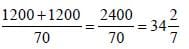SSC CGL Previous Year Questions: Average- 2 - Question 15

On a journey across Kolkata, a taxi averages 50 km per hour for 50% of the distance. 40 km per hour for 40% of it and 20 km per hour for the remaining. The average speed in km/hour, for the whole journey is :        (SSC Sub. Ins. 2013)

Detailed Solution for SSC CGL Previous Year Questions: Average- 2 - Question 15

Total distance = 100 km.
Total time =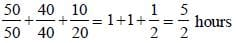∴ Average speed =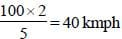SSC CGL Previous Year Questions: Average- 2 - Question 16

The batting average for 30 innings of a cricket player is 40 runs. His highest score exceeds his lowest score by 100 runs. If these two innings are not included, the average of the remaining 28 innings is 38 runs. The lowest score of the player is:       (SSC Sub. Ins. 2013)

Detailed Solution for SSC CGL Previous Year Questions: Average- 2 - Question 16

Lowest score = x
Highest score = x + 100
∴ 28 × 38 + x + x + 100 = 30 × 40
⇒ 1064 + 2x + 100 = 1200
⇒ 2x = 1200 – 1164 = 36
⇒ x = 18

SSC CGL Previous Year Questions: Average- 2 - Question 17

Average of first five prime numbers is       (SSC Multitasking 2013)

Detailed Solution for SSC CGL Previous Year Questions: Average- 2 - Question 17

First five prime numbers are 2, 3, 5, 7, 11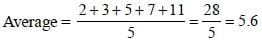SSC CGL Previous Year Questions: Average- 2 - Question 18

Out of 20 boys, 6 are each of 1 m 15 cm height, 8 are of 1 m 10 cm and rest of 1 m 12 cm. The average height of all of them is      (SSC Multitasking 2013)

Detailed Solution for SSC CGL Previous Year Questions: Average- 2 - Question 18

Average height =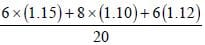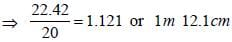SSC CGL Previous Year Questions: Average- 2 - Question 19

The average of 20 numbers is 15 and the average of first five is 12. The average of the rest is       (SSC CGL 2nd Sit. 2013)

Detailed Solution for SSC CGL Previous Year Questions: Average- 2 - Question 19

If the average of remaining numbers be x, then
20 × 15 = 5 × 12 + 15x
⇒ 300 = 60 + 15x
⇒ 15x = 300 – 60 = 240
⇒ x = 240/15 = 16.

SSC CGL Previous Year Questions: Average- 2 - Question 20

If a, b, c, d, e are five consecutive odd numbers, their average is        (SSC CGL 2nd Sit. 2013)

Detailed Solution for SSC CGL Previous Year Questions: Average- 2 - Question 20

b = a + 2
c = b + 2 = a + 4
d = c + 2 = a + 6
e = d + 2 = a + 8
∴ Required average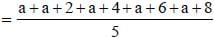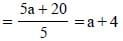SSC CGL Previous Year Questions: Average- 2 - Question 21

The average of the first five multiples of 7 will be        (SSC CGL 2nd Sit. 2013)

Detailed Solution for SSC CGL Previous Year Questions: Average- 2 - Question 21

AverageSSC CGL Previous Year Questions: Average- 2 - Question 22

Out of 40 boys in a class, average weight of 30 is 60 kg and the average weight of the remaining is 56 kg. The average weight (in kilogram) of the whole class is        (SSC CGL 2st Sit. 2013)

Detailed Solution for SSC CGL Previous Year Questions: Average- 2 - Question 22

Average weight of 30 = 60 kg
⇒ Sum of weight of 30 boys = 1800
Average weight of 10 =56 kg
⇒ Sum of weight of 10 boys = 560
Average weight of the whole class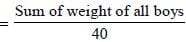sum of weight of 30 boys +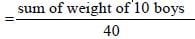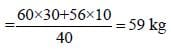SSC CGL Previous Year Questions: Average- 2 - Question 23

The average of the first nine integral multiples of 3 is        (SSC CGL 1st Sit. 2013)

Detailed Solution for SSC CGL Previous Year Questions: Average- 2 - Question 23

Required average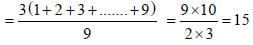SSC CGL Previous Year Questions: Average- 2 - Question 24

The average of nine numbers is 50. The average of the first five numbers is 54 and that of the last three numbers is 52.Then the sixth number is       (SSC CGL 1st Sit. 2013)

Detailed Solution for SSC CGL Previous Year Questions: Average- 2 - Question 24

The sixth number = 9 × 50 – 5 × 54 – 3 × 52
= 450 – 270 – 156 = 24

SSC CGL Previous Year Questions: Average- 2 - Question 25

A cricket player after playing 10 tests scored 100 runs in the 11th test. As a result, the average of his runs is increased by 5. The present average of runs is      (SSC CGL 1st Sit. 2013)

Detailed Solution for SSC CGL Previous Year Questions: Average- 2 - Question 25

If the average in 10 tests be x, then,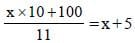x × 10 + 100 = (x + 5) × 11
⇒ 11x – 10x = 100 – 55
⇒ x = 45
∴ Required average = 50

SSC CGL Previous Year Questions: Average- 2 - Question 26

The average of 50 numbers is 38. If two numbers namely 45 and 55 are discarded, the average of the remaining numbers is :         (SSC CGL 1st Sit. 2013)

Detailed Solution for SSC CGL Previous Year Questions: Average- 2 - Question 26

New average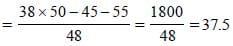SSC CGL Previous Year Questions: Average- 2 - Question 27

Out of 10 teachers of a school, one teacher retires and in his place, a new teacher of age 25 years joins. As a result, average age of teachers is reduced by 3 years. The age (in years) of the retired teacher is :       (SSC CGL 1st Sit. 2013)

Detailed Solution for SSC CGL Previous Year Questions: Average- 2 - Question 27

Age of retired teacher = 25 + 3 × 10 = 55 years

SSC CGL Previous Year Questions: Average- 2 - Question 28

The average weight of 12 crewmen in a boat is increased by 1/3 kg, when one of the crewmen whose weight is 55 kg is replaced by a new man. What is the weight of that new men ?            (SSC CHSL 2012)

Detailed Solution for SSC CGL Previous Year Questions: Average- 2 - Question 28

Short-cut method:
Weight of new crewmen = Replaced man's weight + [No. of crew men × increment in average]
= 55 + 12 x (1/3) = 59 kg.

SSC CGL Previous Year Questions: Average- 2 - Question 29

The average of four consecutive even numbers is 9. Find the largest number.           (SSC CHSL 2012)

Detailed Solution for SSC CGL Previous Year Questions: Average- 2 - Question 29

Let the consecutive even numbers are 2n, 2n + 2,
2n + 4 and 2n + 6
Average =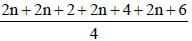8n + 12 = 4 × 9 ⇒ n = 3
Hence, the numbers are 6, 8, 10 and 12. Largest among them is 12.

SSC CGL Previous Year Questions: Average- 2 - Question 30

A batsman in his 12th innings makes a score of 63 runs and there by increases his average scores by 2. What is his average after the 12th innings?           (SSC CHSL 2012)

Detailed Solution for SSC CGL Previous Year Questions: Average- 2 - Question 30

Let the average of batsman after 11th innings = A
Total score made by batsman at the end of 11th innings/11 = A
∴ Total score after 11th innings = 11 A
Now, (Total score after 11th innings + score made in 12th innings)/12 = A + 2
⇒ 11A + 63 = (A + 2) × 12
⇒ 11A – 12 A = 24 – 63
⇒ A = 39
12th innings average = 39 + 2 = 41

SSC CGL Previous Year Questions: Average- 2 - Question 31

The average of 5 consecutive numbers is n. If the next two numbers are also included, the average of the 7 numbers will       (SSC CGL 2nd Sit. 2012)

Detailed Solution for SSC CGL Previous Year Questions: Average- 2 - Question 31

Let the numbers be n – 2, n – 1, n, n + 1 and n + 2.
Their average = n.
Next two consecutive numbers are n + 3 and n + 4.
Therefore the average of 7 consecutive numbers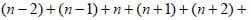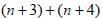/7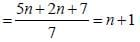SSC CGL Previous Year Questions: Average- 2 - Question 32

The average age of a jury of 5 is 40. If a member aged 35 resigns and a man aged 25 becomes a member, then the average age of the new jury is      (SSC CGL 2nd Sit. 2012)

Detailed Solution for SSC CGL Previous Year Questions: Average- 2 - Question 32

Required average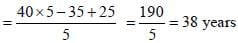SSC CGL Previous Year Questions: Average- 2 - Question 33

The mean of 19 observation is 24. If the mean of the first 10 observations is 17 and that of the last 10 observations is 24, find the 10th observation.       (SSC CGL 1st Sit.  2012)

Detailed Solution for SSC CGL Previous Year Questions: Average- 2 - Question 33

10th observation
= 24 × 10 + 17 × 10 – 19 × 24
= 240 + 170 – 456 = – 46

SSC CGL Previous Year Questions: Average- 2 - Question 34

The average temperature of Monday, Tuesday and Wednesday was 30°C and that of Tuesday, Wednesday and Thursday was 33°C. If the temperature on Monday was 32°C, then the temperature on Thursday was:       (SSC CGL 2012)

Detailed Solution for SSC CGL Previous Year Questions: Average- 2 - Question 34

M + T + W = 90° ...(i)
T + W + Th = 99° ...(ii)
By equation (ii) – (i)
Th – M = 9° ⇒ Th – 32° = 9°
⇒ Th = 9° + 32° = 41°

SSC CGL Previous Year Questions: Average- 2 - Question 35

If the average of  39, 48, 51, 63, 75, 83, x and 69 is 60, then the value of x is       (SSC CGL 1st Sit.  2012)

Detailed Solution for SSC CGL Previous Year Questions: Average- 2 - Question 35

39 + 48 + 51 + 63 + 75 + 83 + x + 69 = 60 × 8
⇒ 428 + x = 480
⇒ x = 480 – 428 = 52

SSC CGL Previous Year Questions: Average- 2 - Question 36

The average age of four boys, five years ago was 9 years. On including a new boy, the present average age of all the five is 15 years. The present age of the new boy is       (SSC CGL 1st Sit. 2012)

Detailed Solution for SSC CGL Previous Year Questions: Average- 2 - Question 36

Sum of the present ages of four boys = 9 × 4 + 20 = 56 years
Sum of the present ages of five boys
= 15 × 5 = 75 years
∴ Present age of new boy
= 75 – 56 = 19 years

SSC CGL Previous Year Questions: Average- 2 - Question 37

The average of 18 observations is recorded as 124. Later it was found that two observations with values 64 and 28 were entered wrongly as 46 and 82. Find the correct average of the 18 observations.        (SSC CGL 2nd Sit. 2011)

Detailed Solution for SSC CGL Previous Year Questions: Average- 2 - Question 37

Difference in observations = 64 + 28 – 46 – 82 = –36
∴ Correct average = 124 - (36/18) = 122

SSC CGL Previous Year Questions: Average- 2 - Question 38

The average of three consecutive odd numbers is 12 more than one third of the first of these numbers. What is the last of the three numbers?       (SSC CGL 1st Sit. 2011)

Detailed Solution for SSC CGL Previous Year Questions: Average- 2 - Question 38

Let 3 consecutive odd no. be x, x + 2 and x + 4
ATQ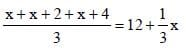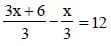⇒ 2x + 6 = 36 ⇒ x = (36-6)/2 = 15
last no = 15 + 4 = 19

SSC CGL Previous Year Questions: Average- 2 - Question 39

The mean of 50 numbers is 30. Later it was discovered that two entries were wrongly entered as 82 and 13 instead of 28 and 31. Find the correct mean.        (SSC CGL 1st Sit. 2011)

Detailed Solution for SSC CGL Previous Year Questions: Average- 2 - Question 39

Required average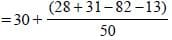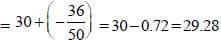SSC CGL Previous Year Questions: Average- 2 - Question 40

The average of odd numbers upto 100 is       (SSC CGL 2nd Sit. 2010)

Detailed Solution for SSC CGL Previous Year Questions: Average- 2 - Question 40

Average of first n natural odd numbers = n
No. of odd numbers upto 100 = 50
Sum of 50 odd numbers = 502
Average =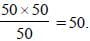## SSC CGL (Tier - 1) - Previous Year Papers (Topic Wise)

250 tests
Information about SSC CGL Previous Year Questions: Average- 2 Page
In this test you can find the Exam questions for SSC CGL Previous Year Questions: Average- 2 solved & explained in the simplest way possible. Besides giving Questions and answers for SSC CGL Previous Year Questions: Average- 2, EduRev gives you an ample number of Online tests for practice

250 tests